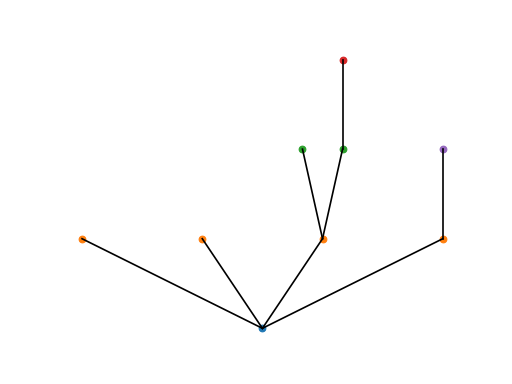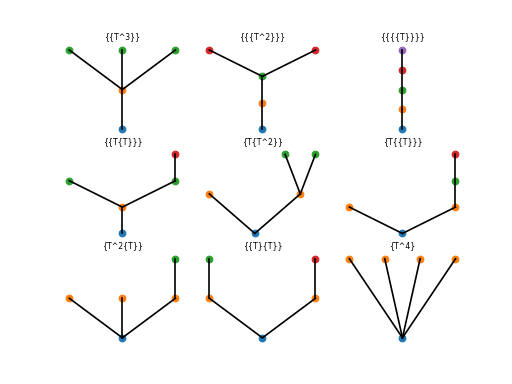# Rooted Trees¶class nodepy.rooted_trees.RootedTree(strg)[source]

A rooted tree is a directed acyclic graph with one node, which has no incoming edges, designated as the root. Rooted trees are useful for analyzing the order conditions of multistage numerical ODE solvers, such as Runge-Kutta methods and other general linear methods.

The trees are represented as strings, using one of the notations introduced by Butcher (the third column of Table 300(I) of Butcher’s text). The character ‘T’ is used in place of $\tau$ to represent a vertex, and braces ‘{ }’ are used instead of brackets ‘[ ]’ to indicate that everything inside the braces is joined to a single parent node. Thus the first four trees are:

‘T’, ‘{T}’, ‘{T^2}’, {{T}}’

These can be generated using the function list_trees(), which returns a list of all trees of a given order:

>>> from nodepy import *
>>> for p in range(4): print(rt.list_trees(p))
['']
['T']
['{T}']
['{{T}}', '{T^2}']


Note that the tree of order 0 is indicated by an empty string.

If the tree contains an edge from vertex A to vertex B, vertex B is said to be a child of vertex A. A vertex with no children is referred to as a leaf.

Warning

One important convention is assumed in the code; namely, that at each level, leaves are listed first (before any other subtrees), and if there are $n$ leaves, we write ‘T^n’.

Note

Currently, powers cannot be used for subtrees; thus

‘{{T}{T}}’

is valid, while

‘{{T}^2}’

is not. This restriction may be lifted in the future.

Examples:

>>> from nodepy import rooted_trees as rt
>>> tree=rt.RootedTree('{T^2{T{T}}{T}}')
>>> tree.order()
9
>>> tree.density()
144
>>> tree.symmetry()
2


Topologically equivalent trees are considered equal:

>>> tree2=RootedTree('{T^2{T}{T{T}}}')
>>> tree2==tree
True


We can generate Python code to evaluate the elementary weight corresponding to a given tree for a given class of methods:

>>> rk.elementary_weight_str(tree)
'dot(b,dot(A,c)*dot(A,c*dot(A,c))*c**2)'

References:
TODO: - Check validity of strg more extensively
• Accept any leaf ordering, but convert it to our convention
• convention for ordering of subtrees?

## Plotting trees¶

A single tree can be plotted using the plot method of the RootedTree class. For convenience, the method plot_all_trees() plots the whole forest of rooted trees of a given order.

RootedTree.plot(nrows=1, ncols=1, iplot=1, ttitle='')[source]

Plots the rooted tree.

INPUT: (optional)
• nrows, ncols – number of rows and columns of subplots in the figure
• iplot – index of the subplot in which to plot this tree

These are only necessary if plotting more than one tree in a single figure using subplot.

OUTPUT: None.

The plot is created recursively by plotting the root, parsing the subtrees, plotting the subtrees’ roots, and calling _plot_subtree on each child

from nodepy.rooted_trees import *
tree=RootedTree('{T^2{T{T}}{T}}')
tree.plot()from nodepy.rooted_trees import *
plot_all_trees(5)## Functions on rooted trees¶

RootedTree.order()[source]

The order of a rooted tree, denoted $r(t)$, is the number of vertices in the tree.

Examples:

>>> from nodepy import rooted_trees as rt
>>> tree=rt.RootedTree('{T^2{T{T}}}')
>>> tree.order()
7

RootedTree.density()[source]

The density of a rooted tree, denoted by $\gamma(t)$, is the product of the orders of the subtrees.

Examples:

>>> from nodepy import rooted_trees as rt
>>> tree=rt.RootedTree('{T^2{T{T}}}')
>>> tree.density()
56


Reference:

RootedTree.symmetry()[source]

The symmetry $\sigma(t)$ of a rooted tree is...

Examples:

>>> from nodepy import rooted_trees as rt
>>> tree=rt.RootedTree('{T^2{T{T}}}')
>>> tree.symmetry()
2


Reference:

## Computing products on trees¶

RootedTree.Gprod(alpha, beta, alphaargs=[], betaargs=[])[source]

Returns the product of two functions on a given tree.

INPUT:
alpha, beta – two functions on rooted trees
that return symbolic or numeric values
alphaargs – a string containing any additional arguments
that must be passed to function alpha
betaargs – a string containing any additional arguments
that must be passed to function beta
OUTPUT:
(alpha*beta)(self) i.e., the function that is the product (in G) of the functions alpha and beta. Note that this product is not commutative.

The product is given by

$(\alpha*\beta)(‘’)=\beta(‘’)$

$(\alpha*\beta)(t) = \lambda(\alpha,t)(\beta) + \alpha(t)\beta(‘’)$

Note

Gprod can be used to compute products of more than two functions by passing Gprod itself in as beta, and providing the remaining functions to be multiplied as betaargs.

Examples:

>>> from nodepy import rt
>>> tree = rt.RootedTree('{T{T}}')
>>> tree.Gprod(rt.Emap,Dmap)
1/2


Reference: [butcher2003] p. 276, Thm. 386A

RootedTree.lamda(alpha, extraargs=[])[source]

Computes Butcher’s functional lambda on a given tree for the function alpha. This is used to compute the product of two functions on trees.

INPUT:

• alpha – a function on rooted trees
• extraargs – a list containing any additional arguments that must be passed to alpha
OUTPUT:
• tprod – a list of trees [t1, t2, ...]
• fprod – a list of numbers [a1, a2, ...]

The meaning of the output is that $\lambda(\alpha,t)(\beta)=a1*\beta(t1)+a2*\beta(t2)+...$

Examples:

>>> from nodepy import rt
>>> tree = rt.RootedTree('{T{T}}')
>>> tree.lamda(rt.Emap)
(['T', '{T}', '{{T}}', '{T}', '{T^2}', '{T{T}}'], [1/2, 1, 1, 1/2, 1, 1])

Reference:
[butcher2003] pp. 275-276